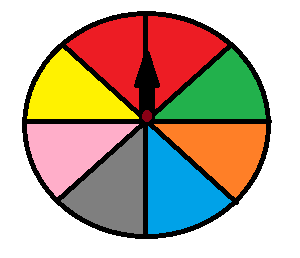# Probability Problems AND/OR Worksheets

Probability Problems That Include AND/OR - The probability problems are considered as one of the most difficult areas of mathematics. Given that, the solutions are apparently approximative and rarely definite. By definition, the probability is defined as the chance that the event will happen. Now, there are two types of probability questions, let us discuss each of them in detail. Firstly, if there are two independent events, then we multiply the probability of the first event with the probability of the other. For instance, if there are three dice, then what is the probability of getting three 5s. The probability of getting a single 5 is 1/6, then the probability of getting 3 - 5s is 1/ 6× 1/6 × 1/6= 1/216. This kind of question adds AND to the mix; hence, P (5 AND 5 AND 5). Secondly, if we want to find the probability of the mutually exclusive events, we use OR between the two events. For instance, there is a wheel of fortune.Now, in this scenario, you get a prize if your arrow ends up on a red or pink field. What is the probability that your arrow will end up in red or pink? P(red)= 2/8 = 1/4, P(pink)= 1/8, P(pink or red)= 1/8 + 1/4 = 3/8.

• ### Basic Lesson

Guides students through solving Probability Problems AND/OR. What is probability of getting number 1 or 4 when dice numbered from 1 to 6 is rolled?

• ### Intermediate Lesson

Demonstrates the concept of advanced skill while solving probability problems that include the And/Or concepts. If you randomly choose a letter in the word "HIGHEST", find the probability that you choose a T or H. Since the word contains 1 T, it means T has one possibility to be selected out of seven. Since the word contains 2 H, it means H has two possibilities to be selected out of seven.

• ### Independent Practice 1

A really great activity for allowing students to understand the concepts of the probability problems that include the And/Or concepts. Jack has to select a pen and a pencil from 6 different pencils and blue, black, green and red colored pens. Find the probability of selecting a green colored pen and any of the given pencil.

• ### Independent Practice 2

Students use Probability Problems AND/OR in 20 assorted problems. The answers can be found below.

• ### Homework Worksheet

Students are provided with 12 problems to achieve the concepts of probability problems that include the And/Or concepts.

• ### Skill Quiz

This tests the students ability to understand probability problems that include the And/Or concepts.